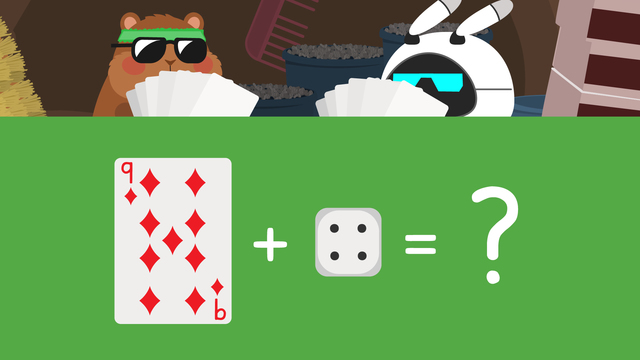Rating

Ø 3.5 / 6 ratings
The authorsTeam Digital
CCSS.MATH.CONTENT.1.OA.C.5

## Basics on the topicCounting to Add

Whenever you combine two numbers to find the answer, you do something called addition in math. In this text, you can learn how to find the sum of numbers using the counting on addition strategy.

## Addition – Counting On Strategy

One way to add numbers together is to count on. We use counting on by starting with one number in the addition problem and then counting on the amount of the second number. It's easier to start with the larger number and count on with the smaller one. The number we stop on is the answer! To help count, we can use our fingers or draw dots!

## Counting On Strategy – Example

Let's try it with the example six plus two. We will start at six since it is the larger number.The plus means we are adding so we need to count forward. The second number is two so we count forward two numbers. We will use the dots on the die to help, but you could always use your fingers or draw out two dots.We count two times from six: seven, eight! Eight is the number we stopped on, so we write it next to the equal sign. This means six plus two equals eight!## Counting On in Addition – Summary of Steps

To practice this strategy, you can try counting on cards for addition, counting on dice, or both! When adding two numbers, we can count on to solve.

Step # What to do
1 Start at the larger number.
2 Count on the second number.
3 Use your fingers or draw dots
to help count the numbers out.
4 The number you stop on is the answer.
You write the answer next to the equal sign.

Have you practiced with counting on addition worksheets yet? On this website, you can also find first grade counting on addition worksheets as well as interactive exercises and other activities with which you can practice the counting on method.

Would you like to apply the knowledge you’ve learned? You can review and practice it with the tasks for the video Counting to Add.
• ### Find the sum of 4 + 2 by counting on from 4

Hints

Use your fingers to count on.

• First, select the bigger number. The bigger number here is 6.
• Then, count on by adding the smaller number. The smaller number here is 2.
• You can count on by adding 6 + 2 = 8.
Solution

4 + 2 = 6

• ### Find the sum of each problem by counting on.

Hints

Use your fingers to count on.

• First, select the bigger number. The bigger number here is 9.
• Then, count on by adding the smaller number. The smaller number here is 4.
• You can count on by adding 9 + 4 = 13.
Solution
• 5 + 3 = 8
• 2 + 2 = 4
• 7 + 3 = 10
• 9 + 5 = 14
• ### Count on to find the sum of each addition problem.

Hints

• First, select the bigger number. The bigger number here is 6.
• Then, count on by adding the smaller number. The smaller number here is 2.
• You can count on by adding 6 + 2 = 8.
Solution
• 4 + 3 = 7
• 9 + 2 = 11
• 8 + 5 = 13
• 7 + 4 = 11
• ### Find the sum of each addition problem by counting on.

Hints

Use your fingers to count on.

• First, select the bigger number. The bigger number here is 6.
• Then, count on by adding the smaller number. The smaller number here is 2.
• You can count on by adding 6 + 2 = 8.
Solution
• 6 + 3 = 9
• 10+ 2 = 12
• 5 + 2 = 7
• 8 + 3 = 11
• ### Solve 5 + 5 by counting on.

Hints

Use your fingers to count on.

• Since both numbers are the same, it does not matter which one you start with when counting on.
• You can count on by adding 6 + 6 = 12.

5 + 5 does not equal 9.

Solution

5 + 5 = 10

Hints